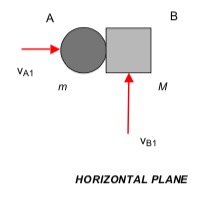# Homework H.4.PAnimationHINTS:

STEP 1 - FBD: Draw three free body diagrams (FBDs): A, B and A+B.
STEP 2 - Kinetics:  From the FBD of A, we know that it will continue to move along the same line of travel. In addition, the FBD of B shows that the t-component of velocity for B will remain unchanged, where the "t"-direction is tangent to the plane of contact of A and B. In the n-direction (perpendicular to the t-direction), you have both the linear-impulse equation and the coefficient of restitution equation.
STEP 3 - Kinematics
STEP 4 - Solve: Solve for M and from your two kinetics equations above.

COMMENT: This problem is posed in a somewhat "backward" way as compared to many problems in impact. Typically mass and COR parameters are given, and we solve for final velocities. Here we are given some information on the final velocities, and we are asked to find mass and COR values. You use the same equations for either type of problem, you just solve these equations in a different way.

## 3 thoughts on “Homework H.4.P”

1.Bailey Joseph Barton says:

Why is it that the blocks do not stick together and move as one system after colliding?

1.CMK says:

Sticking requires a coefficient of restitution of e = 0. Since they do not stick, then the simulation suggests that your answer for e should not be zero.

2.Sung Namgung says:

I think it is because they have different masses as well have different velocities.